# Welcome to pyrodeo!¶

Pyrodeo is a Python implementation of RODEO (ROe solver for Disc-Embedded Objects), a Roe solver implementation aimed at hydrodynamic simulations of astrophysical discs.

## Installation¶

If Python is not installed, download from here and install. The latest versions of Python come with package manager pip included. Then Pyrodeo can be installed from the command line simply by entering:

pip install pyrodeo


## Quick start¶

Within Python, first import the simulation module:

>>> import pyrodeo


Create a simulation in Cartesian geometry with standard parameters:

>>> sim = pyrodeo.Simulation.from_geom('cart')


Run the simulation up to t=0.25:

>>> sim.evolve([0.25])


Since the standard initial conditions consist of constant density and pressure and zero velocity, no visible evolution takes place. For more interesting examples, see Examples.

## Equations solved¶

The current version supports inviscid isothermal hydrodynamics in three spatial dimensions. Isothermal means the pressure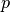is related to the density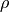simply through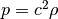, where the sound speed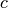is either a constant (fully isothermal) or a prescribed function of position (locally isothermal). Four geometries are available: Cartesian coordinates, the shearing sheet, cylindrical coordinates and spherical coordinates.

### Cartesian coordinates¶

In Cartesian coordinates, we have the continuity equation: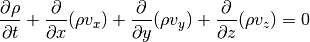Momentum in x-direction: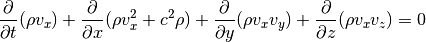Momentum in y-direction: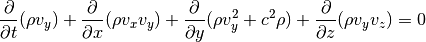Momentum in z-direction: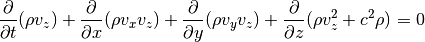### Shearing Sheet¶

The Shearing Sheet is essentially a Cartesian model of a small patch in an astrophysical disc. This patch is rotating at the local Keplerian angular velocity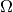, which means that Coriolis and centrifugal-type forces need to be included on the right-hand side of the equations. On the other hand, the patch is assumed to be small enough so that a local Cartesian frame can be used in stead of cylindrical coordinates. Usually the computational domain is taken to be periodic in y and shear-periodic in x (periodic but corrected for the shear). We therefore still have the continuity equation:The x-momentum equation now includes source terms on the right-hand side: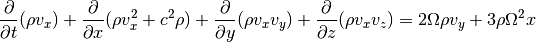Same for the momentum equation in y-direction: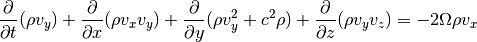In the z-direction we get a source term due to the vertical component of the stellar gravity: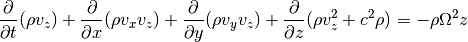Note

In the shearing sheet the sound speed should really be constant (no locally isothermal shearing sheet). Together, sound speed and angular velocity define a length scale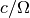, which is a measure of the scale height of the disc. Typically one chooses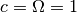, so that distances are measured in scale heights and time in inverse orbital frequency.

### Cylindrical coordinates¶

For a full disc in two dimensions cylindrical coordinates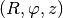are preferred. This time we have geometrical source terms and gravity from the central object to worry about. The continuity equation now reads: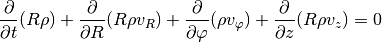The radial momentum equation now includes source terms representing centrifugal and gravitational forces, in addition to a geometrical pressure source term: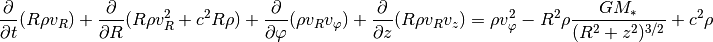In the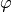direction we get a Coriolis source term: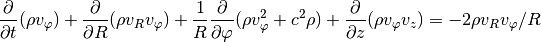In the vertical direction we again have the vertical component of the stellar gravity: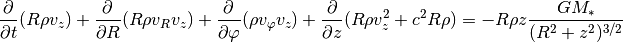Note

The unit of mass is taken to be the mass of the central object. The unit of distance is some reference radius. The unit of time is the inverse angular velocity at the reference radius. In this system of units, the gravitational constant is unity, and one orbit equals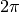time units.

### Spherical coordinates¶

For a full disc in three dimensions spherical coordinates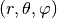are often preferred. The continuity and momentum equations now read: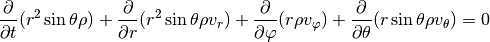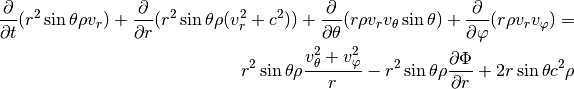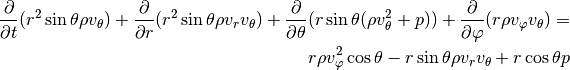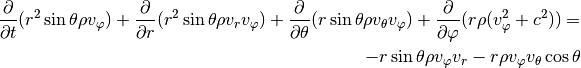### Extra source terms¶

Pyrodeo solves inviscid isothermal hydrodynamics, and in the shearing sheet and cylindrical and spherical geometries only gravity from the central object is considered. Extra physics, as far as it concerns extra source terms, can be added by a user-defined source integration function. See the Examples section. This function is called once per time step and can also be used for monitoring various quantities (mass, torque on planet, etc.).

## Numerical method¶

### Dimensional splitting¶

Pyrodeo uses dimensional splitting to integrate the equations.

#### First direction (x, R, r)¶

For the x direction (therefore neglecting y- and z-derivatives), we can cast the equations into the following form: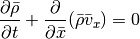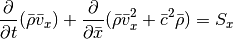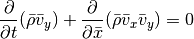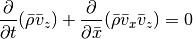For the Cartesian setup, we simply have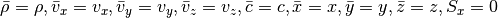For the shearing sheet, we need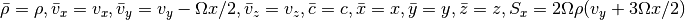In cylindrical coordinates we need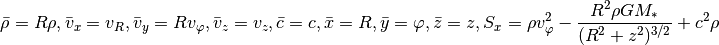Finally, for spherical coordinates we need: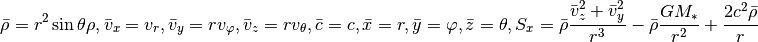#### Second direction (y,)¶

For the y-integration (neglecting x- and z-derivatives) we can cast the equations in the form: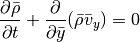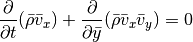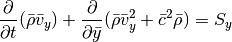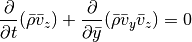For both the Cartesian setup and the shearing sheet, we simply have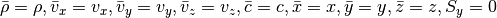In cylindrical coordinates we need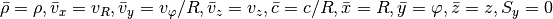Finally, spherical coordinates: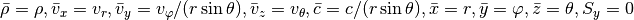#### Third direction (z,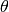)¶

Finally, for the z integration we can cast the equations in the form: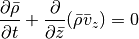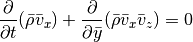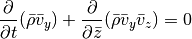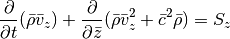For both the Cartesian setup, we simply have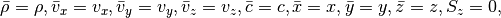with the shearing sheet being exactly the same but with a non-zero source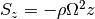.

In cylindrical coordinates we need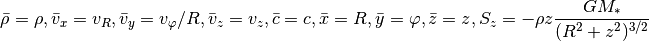Finally, spherical coordinates: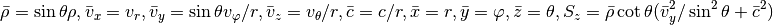#### Unified approach¶

Note that the resulting equations are very symmetric in x, y and z: if we swap x and y in the y integration the equations have exactly the same form as for the x integration. Similar for the z integration when swapping z and x. Therefore, if we prepare all quantities appropriately, we only need a single hydrodynamic solver that is able to advance the systemThis is what is done in the Roe class. The necessary preparation is done in the Hydro class.

In the case of the shearing sheet and the cylindrical disc there is a large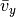that severely limits the time step. This limit can be overcome by splitting the y integration one more by writing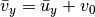where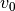is independent of y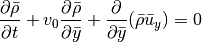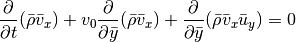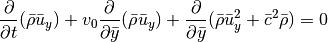The terms involvingmake up a linear advection problem that can be solved straightforwardly for any time step. This is done in the LinearAdvection class. The remaining terms are integrated in the Roe class, but at a much larger time step because presumably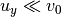.

### Algorithm overview¶

A single time step in Hydro.evolve consists of the following steps:

1. Calculate time step using Hydro.calc_time_step.
2. Set shear periodic boundary conditions if necessary.
3. Preprocessing step to cast the equations in the same form for all geometries and directions, while at the same time calculating the source term using Hydro.preprocess.
4. Use the Roe solver to advance the hydrodynamic equations using Roe.step.
5. Do orbital advection if necessary using Hydro.orbital_advection.
6. Do the inverse of step 3, getting all quantities back to their original form in Hydro.postprocess.
7. Integrate any extra source terms.

### Boundary conditions¶

The available boundary conditions are

• ‘closed’: closed boundary, i.e. no mass flow through the boundary. Waves will be reflected off the boundary.
• ‘periodic’: periodic boundary.
• ‘nonreflecting’: allow waves to pass through unhindered.
• ‘symmetric’: assume boundary is a symmetry plane. Very much like a closed boundary, but less general in that it needs the boundary to be a plane of symmetry.

By default, all boundaries are set to be closed; this can be changed by changing the boundaries attribute of the Param class.

## Output¶

Once the integration routine Simulation.evolve has finished the final state is available through simulation.state(). In addition, an output file rodeo.h5 is created containing the state at all specified checkpoints. This is an HDF5 file created with h5py. It contains the following groups:

Note

Both state and coordinate arrays include two ghost zones on each side in all directions. This is in order to be able to restore a simulation from a checkpoint.

Note

The value stored in state.vely is the y-velocity with the orbital advection velocity removed! In other words, the equilibrium solution in a constant pressure shearing sheet or cylindrical disc has vanishing state.vely.

An example of reading the file and plotting using matplotlib:

#!/usr/bin/python

import numpy as np
import matplotlib.pyplot as plt
import h5py

with h5py.File('./rodeo.h5', "r") as hf:
# Select last available checkpoint
last_checkpoint = None
for k in hf.keys():
if (k != 'coords' and k != 'param'):
last_checkpoint = k

# Get x coordinate
gc = hf.get('coords')
x = np.array(gc.get('x'))

# Get density
g = hf.get(last_checkpoint)
dens = np.array(g.get('dens'))
# Simulation time at checkpoint
t = g.attrs['time']

print('Plotting ' + last_checkpoint + ' at t = {}'.format(t))

plt.plot(x[:,0], np.mean(dens, axis=1))

plt.show()


## Examples¶

### Shock tube¶

A simple example in Cartesian geometry is a one-dimensional isothermal shock tube:

#!/usr/bin/python

import numpy as np
import matplotlib.pyplot as plt
import pyrodeo

# Create simulation with default resolution and domain
sim = pyrodeo.Simulation.from_geom('cart')

# The basic state will have density and sound speed unity everywhere,
# and the velocity will be zero. In order to create a simple shock tube,
# now set density to 1/10 for x > 0.
sel = np.where(sim.coords.x > 0.0)
sim.state.dens[sel] = 0.1

# Evolve until t = 0.25
sim.evolve([0.25], new_file=True)

# Plot results
plt.plot(sim.coords.x[:,0,0], sim.state.dens[:,0,0])
plt.show()


The standard grid dimensions are (100,1), which means 100 cells in x and 1 in y. Try a higher resolution by explicitly specifying the dimensions in Simulation.from_geom.

### Instability in shearing sheet¶

A more demanding two-dimensional calculation involves the instability of a sharp density ridge in the shearing sheet:

#!/usr/bin/python

import numpy as np
import pyrodeo

# Domain half-width in x and y
Lx = 2.0
Ly = 20.0

# Create simulation, setting grid dimensions and domain
sim = pyrodeo.Simulation.from_geom('sheet',
dimensions=[32, 64, 1],
domain=([-Lx, Lx], [-Ly, Ly], []))
sim.param.boundaries = ['shear periodic','shear periodic']
sim.param.boundaries = ['periodic','periodic']

# Density profile: single maximum in middle of domain
sim.state.dens = 0.5*np.cos(np.pi*sim.coords.x/Lx) + 1.0
# Equilibrium vy to compensate for pressure gradient
sim.state.vely = -0.25*np.pi*np.sin(np.pi*sim.coords.x/Lx)/(sim.state.dens*Lx)
# Add some noise to seed instability
sim.state.dens += 0.01*np.random.random_sample(np.shape(sim.state.dens))

# Evolve until t = 100.0
sim.evolve(0.25*np.arange(400), new_file=True)


The checkpoints have been chosen close enough together to allow for the results to be animated:

#!/usr/bin/python

import numpy as np
import matplotlib.pyplot as plt
import matplotlib.animation as animation
import h5py

# Return density at checkpoint n
def density_at_checkpoint(file_name, n):
dens = None
with h5py.File(file_name, 'r') as hf:
maxn = len(hf.keys()) - 2
if n >= maxn:
n = maxn - 1

s = "checkpoint{}".format(n)
g = hf.get(s)
dens = np.array(g.get('dens'))

return dens

fig = plt.figure()

# Show initial conditions (checkpoint 0)
file_name = './rodeo.h5'
dens = density_at_checkpoint(file_name, 0)
im = plt.imshow(dens[:,:,0], animated=True)

# Get next checkpoint
n = 0
def updatefig(*args):
global n

dens = density_at_checkpoint(file_name, n)
im.set_array(dens[:,:,0])
n += 1

return im,

# Animate!
ani = animation.FuncAnimation(fig, updatefig, interval=50, blit=True)
plt.show()


### Disc-planet interaction¶

As the final, most complex example, consider a planet embedded in a disc in cylindrical coordinates. Since pyrodeo only includes gravity from the central star, we need to provide an extra source term to account for the gravitational force due to the planet. In addition, we define wave-killing zones on the radial edges of the domain to avoid wave reflection. It will take some time to run this simulation, so have a cup of tea and come back to see a Jupiter-like planet carve out a gap in the disc.

#!/usr/bin/python

import numpy as np
import pyrodeo

# Extra source terms: planet gravity
def planet_source(t, dt, coords, state, planetParam):
# Mass ratio planet/star
mp = planetParam
# Softening length planet potential
eps = planetParam

# Coordinates
r = coords.x
p = coords.y

# Planet coordinates
rp = 1.0
pp = 0.0

# Distance to the planet
dist = np.sqrt(r*r + rp*rp - 2.0*r*rp*np.cos(p - pp) + eps*eps)

dpotdr = mp*(r - rp*np.cos(p - pp))/(dist*dist*dist)
dpotdp = mp*r*rp*np.sin(p - pp)/(dist*dist*dist)

# Indirect term
dpotdr += mp*np.cos(p - pp)/(rp*rp)
dpotdp -= mp*r*np.sin(p - pp)/(rp*rp)

# Resulting source term
source_velx = -dpotdr
source_vely = -dpotdp/(r*r)

# Damping boundary conditions
Rin = 100.0*(r - 0.5)*(r - 0.5)
Rin[np.where(r > 0.5)] = 0.0
Rout = (r - 2.1)*(r - 2.1)/(0.4*0.4)
Rout[np.where(r < 2.1)] = 0.0
R = (Rin + Rout)*np.power(r, -1.5)

# Damp towards initial state
source_dens = -(state.dens - 1.0)*R
source_velx -= state.velx*R
source_vely -= state.vely*R

# Integrate extra source terms
state.dens += dt*source_dens*state.no_ghost
state.velx += dt*source_velx*state.no_ghost
state.vely += dt*source_vely*state.no_ghost

sim = pyrodeo.Simulation.from_geom('cyl',
dimensions=[128, 384, 1],
domain=([0.4, 2.5], [-np.pi, np.pi], []))

# Sound speed constant H/r = 0.05
sim.state.soundspeed = 0.05*sim.state.soundspeed/np.sqrt(sim.coords.x)
sim.param.boundaries = ['closed','closed']
sim.param.boundaries = ['periodic','periodic']

# Simulate a Jupiter planet up to 100 orbits
sim.evolve(2.0*np.pi*np.array([1.0,2.0,5.0,10.0,20.0,50.0,100.0]),
planet_source, (0.001, 0.6*0.05), new_file=True)


# Class reference¶

## Coordinates¶

Coordinate class used in pyrodeo.

The Coordinate class hold the x, y and z coordinates as 3D ndarrays. In addition, it holds the step size in x, y and z and the size of the grid in x, y and z.

class pyrodeo.coords.Coordinates(x, y, z, log_radial=False)[source]

Class containing coordinates used in pyrodeo.

Parameters: x (ndarray) – 2D ndarray containing x coordinates y (ndarray) – 2D ndarray containing y coordinates z (ndarray) – 2D ndarray containing z coordinates log_radial (bool, optional) – Flag whether x is a logarithmic radial coordinate.

Note

The validity of the arrays is not checked! To be used in a simulation, they should have the same shape, with x[:,j,k] containing the x coordinates for all j,k, y[i,:,k] containing the y coordinates for all i,k and z[i,j,:] containing the z coordinates for all i,j. In addition, x, y and z should have a constant step size.

The following public attributes are available:

x

ndarray – 2D ndarray containing x coordinates

y

ndarray – 2D ndarray containing y coordinates

z

ndarray – 3D ndarray containing z coordinates

dimensions

[int, int, int] – grid dimensions in the x, y and z direction

dxyz

[float, float, float] – step size in the x, y and z direction

log_radial

bool, optional – Flag whether x is a logarithmic radial coordinate.

classmethod from_1d(x, y, z, log_radial=False)[source]

Initialize from 1D arrays.

Build coordinates from existing 1D ndarrays (should include ghost cells). Calculate dimensions and step sizes.

Parameters: x (ndarray) – 1D ndarray containing x coordinates y (ndarray) – 1D ndarray containing y coordinates z (ndarray) – 1D ndarray containing z coordinates log_radial (bool, optional) – Flag whether x is a logarithmic radial coordinate.

Note

The validity of arrays x, y and z is not checked. They should have constant step size.

classmethod from_dims(dimensions=(100, 1, 1), domain=([-0.5, 0.5], [], []), log_radial=False)[source]

Initialize from dimensions and domain size.

Build coordinates given the dimensions of the grid and the size of the domain. Some basic checks are performed to ensure the resulting coordinates are valid.

Parameters: dimensions ((int,int,int), optional) – Dimensions of the grid domain (([float,float],[float,float],[float,float]), optional) – Domain boundaries in x, y and z log_radial (bool, optional) – Flag whether x will be a logarithmic radial coordinate.

## State¶

Definition of State class used in pyrodeo.

The State class holds density, velocity and sound speed for a pyrodeo simulation

class pyrodeo.state.State(dens, velx, vely, velz, soundspeed)[source]

Construct state holding density, velocity and sound speed for a pyrodeo simulation.

Parameters: dens (ndarray) – 3D ndarray containing density. velx (ndarray) – 3D ndarray containing x velocity. vely (ndarray) – 3D ndarray containing y velocity. velz (ndarray) – 3D ndarray containing z velocity. soundspeed (ndarray) – 3D ndarray containing sound speed.

Note

No checks are performed whether density, velocity and sound speed are valid arrays. They should all have the same shape, the same as the arrays of Coordinates.

The following public attributes are available:

dens

ndarray – 3D ndarray containing density.

velx

ndarray – 3D ndarray containing x velocity.

vely

ndarray – 3D ndarray containing y velocity.

velz

ndarray – 3D ndarray containing z velocity.

soundspeed

ndarray – 3D ndarray containing sound speed.

no_ghost

ndarray – 3D ndarray flagging whether a cell is a ghost cell (=0) or an internal cell (=1)

classmethod copy(other_state)[source]

Construct state from other State.

Set this instance of State equal to an other State, performing an explicit copy.

Parameters: other_state (State) – State from which to copy.
classmethod from_dims(dims)[source]

Construct State from grid dimensions.

Construct State given grid dimensions, creating arrays of the correct size with standard (physical) values.

Parameters: dims (int, int, int) – Dimensions of the grid in x, y and z.
swap_velocities(dim)[source]

Swap two velocities.

transpose(axis_order)[source]

Change axis order for all fields.

## Param¶

Simulation parameters used in pyrodeo.

The Param class holds parameters necessary for a pyrodeo simulation.

class pyrodeo.param.Param(param_list)[source]

Create instance from list of parameters.

Parameters: param_list ([str, float, float, float, str, str, str]) – List of parameters; geometry (string), courant (float), fluxlimiter (float), frame_rotation (float), log_radial (bool), boundaries x in (string), boundaries x out (string), boundaries y in (string), boundaries y out (string), boundaries z in (string) and boundaries z out (string)

Note

The validity of the parameters is not checked.

The following public attributes are available:

geometry

string – ‘cart’ (Cartesian coordinates), ‘sheet’ (shearing sheet), ‘cyl’ (cylindrical coordinates) or ‘sph’ (spherical coordinates).

courant

float – Courant number, should be > 0 and < 1.

limiter_param

float – Limiter parameter. Should be between 1 (minmod) and 2 (superbee).

min_dens

float – Minimum density to switch to HLL solver to remain positive.

frame_rotation

float – Frame rotation rate. Ignored in Cartesian coordinates, should be unity in a shearing sheet calculation and corresponds to the angular velocity of the coordinate frame in cylindrical coordinates.

log_radial

bool – Flag whether to use logarithmic radial coordinates in cylindrical geometry.

boundaries

(str,str), (str,str), (str,str) – Boundary conditions (in and out) in x y and z: ‘nonreflecting’, ‘closed’, ‘symmetric’, or ‘periodic’. In shearing sheet mode, the x boundary can be ‘shear periodic’.

classmethod from_geom(geometry, log_radial=False, boundaries=[['closed', 'closed'], ['closed', 'closed'], ['closed', 'closed']])[source]

Initialization from geometry and boundary conditions.

Construct Parameter object from geometry and boundary conditions. All other parameters are set to standard values. Check if geometry and boundary conditions are valid.

Parameters: geometry (string) – ‘cart’ (Cartesian coordinates), ‘sheet’ (shearing sheet), ‘cyl’ (cylindrical coordinates) or ‘sph’ (spherical coordinates). boundaries ((str,str), (str,str), (str,str)) – boundary conditions; ‘closed’, ‘nonreflecting’, ‘symmetric’, or ‘periodic’. In shearing sheet mode, the x boundary can be ‘shear periodic’.
to_list()[source]

Convert parameters to list.

Return list of parameters together with list of types. Used for HDF5 output.

Returns: array of types, list of parameters.

## Conservation law solver¶

Defines a generic conservation law solver.

class pyrodeo.claw_solver.ClawSolver[source]

Generic conservation law solver.

Note

Serves as a base class to construct various solvers. Can not be used on its own.

The following method is available:

limiter(a, b, sb)[source]

Limiter function to limit slopes/fluxes.

This limiter function can, based on the parameter sb, emulate total variation diminishing limiters from minmod (sb = 1) to superbee (sb = 2).

Parameters: a (ndarray) – First slope to compare. b (ndarray) – Second slope to compare. sb (float) – Limiter parameter. Should be >= 1 (minmod, most diffusive limiter) and <= 2 (superbee, least diffusive limiter). Limited slopes. ndarray

Defines a linear advection solver in a periodic domain.

class pyrodeo.linear_advection.LinearAdvection(advection_velocity, limiter_parameter)[source]

Linear advection solver in periodic domain.

Parameters: advection_velocity (ndarray) – Advection velocity. Must have the same shape as State members (density, velocity) to be advected and must be uniform in the first dimension (x). limiter_parameter (float) – Parameter setting the limiter function. Should be >= 1 (minmod limiter, most diffusive) and <= 2 (superbee limiter, least diffusive).

Note

The linear advection equation is solved on a periodic x domain. The advection velocity can not depend on x. If advecting over y, the state will have to be transposed first.

The following public attributes and methods are available:

advection_velocity

ndarray – Advection velocity. Must have the same shape as State members (density, velocity) to be advected and must be uniform in the first dimension (x).

sb

float – Parameter setting the limiter function. Should be >= 1 (minmod limiter, most diffusive) and <= 2 (superbee limiter, least diffusive).

step(dt, dx, state)[source]

Perform one time step dt.

Evolve the linear advection equation over a time step dt, updating the state. The domain can be multidimensional, but evolution is with respect to x. There is no restriction on the magnitude of dt.

Parameters: dt (float) – Time step to take. dx (float) – Step size in x. state (State) – State containing density and velocity

## Roe solver¶

Defines the Roe class defining the Roe solver.

class pyrodeo.roe.Roe(flux_limiter, min_dens)[source]

Construct class for the Roe solver.

Constructor sets two basic attributes (sb and min_dens), after which a time step can be taken through the step() method.

Parameters: flux_limiter (float) – Flux limiter parameter. Should be >= 1 (minmod, most diffusive limiter) and <= 2 (superbee, least diffusive limiter). min_dens (float) – Minimum density when to switch to HLL to preserve positivity.

The following attributes and methods are available:

sb

float – Flux limiter parameter. Should be >= 1 (minmod, most diffusive limiter) and <= 2 (superbee, least diffusive limiter).

min_dens

float – Minimum density when to switch to HLL to preserve positivity.

limit_flux(dens, dens_left, f1dens, f2dens, dtdx)[source]

Limit second order flux to preserve positivity

Calculate the maximum contribution of the second order flux in order for the density to remain positive.

Parameters: dens (ndarray) – Current density. dens_left (ndarray) – Density in the cell to the left f1dens (ndarray) – First order mass flux. f2dens (ndarray) – Second order mass flux. dtdx (float) – Time step / space step Array with values >= 0 and <= 1 specifying the maximum contribution of second order flux for the density to remain positive. ndarray
step(dt, dx, state, source, bc)[source]

Update state for a single time step.

Parameters: dt (float) – Time step. dx (float) – Space step. state (State) – Current State, will be updated. source (ndarray) – Geometric source terms, must have same shape as state.dens. bc (str, str) – Boundary conditions (in and out): ‘periodic’, ‘symmetric’, or ‘closed’ (other boundary conditions are dealt with elsewhere).

## Hydro¶

Defines the Hydro class performing hydrodynamic updates of the state.

class pyrodeo.hydro.Hydro(param, coords)[source]

Construct from existing instances of Param and Coordinates. It constructs a LinearAdvection instance which will deal with orbital advection, and a Roe instance dealing with hydrodynamics of residual velocities.

Parameters: param (Param) – Valid Param object, containing simulation parameters. coords (Coordinates) – Valid Coordinates object, containing x, y and z coordinates. Used to calculate orbital advection velocity.

The following attributes and methods are available:

orbital_advection

LinearAdvection – Instance of LinearAdvection class used to do orbital advection.

roe

Roe – Instance of Roe class dealing with hydrodynamics of residual velocities.

calc_time_step(geometry, coords, state, log_radial=False)[source]

Calculate time step obeying the CFL condition.

Parameters: geometry (str) – ‘cart’, ‘sheet’ or ‘cyl’. coords (Coordinates) – Valid Coordinates object, containing x, y and z coordinates. state (State) – Valid State object, containing density and velocity. log_radial (bool, optional) – Flag indicating whether a logarithmic radial coordinate is used Maximum time step obeying the CFL condition. float
evolve(t, t_max, coords, param, state, source_func, source_param)[source]

Evolve state from t to tmax.

Parameters: t (float) – Current simulation time. t_max (float) – Simulation time to reach before stopping. coords (Coordinates) – Valid Coordinates object, containing x and y coordinates. param (Param) – Valid Param object, containing simulation parameters. state (State) – Valid State object, containing density and velocity. source_func (callable) – Function integrating any extra source terms (non-geometric). It should accept the following arguments: t, dt, coords, state, source_param. source_param (array-like) – Extra parameters for source_func. Tuple consisting of: t (float): new simulation time (= t_max if no problems encountered). State: Updated State. (tuple)
postprocess(coords, param, state, direction)[source]

Inverse of preprocess().

Reverse modifications by preprocess().

Parameters: coords (Coordinates) – Valid Coordinates object, containing x and y coordinates. param (Param) – Valid Param object, containing simulation parameters. state (State) – Valid State object, containing density and velocity. direction (int) – 0 (integrating x) or 1 (integrating y).
preprocess(coords, param, state, direction)[source]

Modify state to quasi-cartesian form and calculate geometric source terms.

Isothermal hydrodynamics allows for a generic form of the equations in all geometries, subject only to modifications in the geometric source terms.

Parameters: coords (Coordinates) – Valid Coordinates object, containing x and y coordinates. param (Param) – Valid Param object, containing simulation parameters. state (State) – Valid State object, containing density and velocity. direction (int) – 0 (integrating x) or 1 (integrating y). Geometric source term of the same shape as state.dens. ndarray
shear_periodic_boundaries(t, coords, state)[source]

Set shear-periodic boundary conditions.

In the shearing sheet geometry, the x direction can be quasi-periodic, i.e. periodic but modified for the shear. Imagine neighbouring sheets shearing past the center sheet.

Parameters: t (float) – Current simulation time. Used to calculate over what distance neighbouring sheets have shifted since t = 0. coords (Coordinates) – Valid Coordinates object, containing x and y coordinates. state (State) – Valid State object, containing density and velocity.

## Simulation¶

Defines the Simulation class holding a pyrodeo simulation

class pyrodeo.simulation.Simulation(param, coords, state, t, direc='./')[source]

Construct pyrodeo simulation.

Construct simulation from existing instances of Param, Coordinates and State and given simulation time.

Parameters: param (Param) – Valid Param object, containing simulation parameters. coords (Coordinates) – Valid Coordinates object, containing x, y and z coordinates state (State) – Valid State object, containing current density, velocity and sound speed. t (float) – Simulation time. direc (str, optional) – Output directory.

Note

While it is possible to set up a simulation using the basic constructor, no checks are performed whether the instances of Param, Coordinates and State are valid. It is safer to use from_geom() which will set up the necessary valid instances.

The following attributes and methods are available:

state

State – State object holding density, velocity and sound speed.

coords

Coordinates – Coordinates object holding x, y and z coordinates.

param

Param – Param object holding simulation parameters.

t

float – Current simulation time.

direc

string – Output directory.

checkpoint(new_file=False)[source]

Save checkpoint in ‘rodeo.h5’

Parameters: new_file (bool,optional) – If true, create new output file ‘rodeo.h5’, otherwise append to file if it exists.
evolve(checkpoints, source_func=None, source_param=None, new_file=False)[source]

Evolve simulation over a list of checkpoints.

Parameters: checkpoints (ndarray) – List of times to save checkpoints. source_func (callable, optional) – Integrate extra source terms. Must be of the form f(t, dt, coords, state, source_param). source_param (ndarray, optional) – Parameters for extra source term. Will be passed to source_func. new_file (bool,optional) – If true, create new output file ‘rodeo.h5’, otherwise append to file if it exists.
classmethod from_checkpoint(direc, n=None)[source]

Constructor for simulation class from checkpoint.

Construct simulation class from previously saved checkpoint.

Parameters: direc (str) – Path to ‘rodeo.h5’. This will be the output directory as well. n (int,optional) – Index of checkpoint to restore. If left None, restore last saved checkpoint.
classmethod from_geom(geometry, dimensions=(100, 1, 1), domain=([-0.5, 0.5], [], []), log_radial=False, direc='./')[source]

Construct simulation from geometry.

Construct simulation class from geometry, grid dimensions and domain size. All other parameters will be set to defaults. Simulation time is set to zero.

Parameters: geometry (string) – ‘cart’ (Cartesian coordinates), ‘sheet’ (shearing sheet), ‘cyl’ (cylindrical coordinates) or ‘sph’ (spherical coordinates). dimensions ((int,int,int),optional) – Grid dimensions in x, y and z. domain ([(float,float),(float,float),(float,float)],optional) – Domain boundaries in x, y and z. log_radial (bool) – Flag whether to use logarithmic radial coordinate direc (str,optional) – Output directory, defaults to current directory.